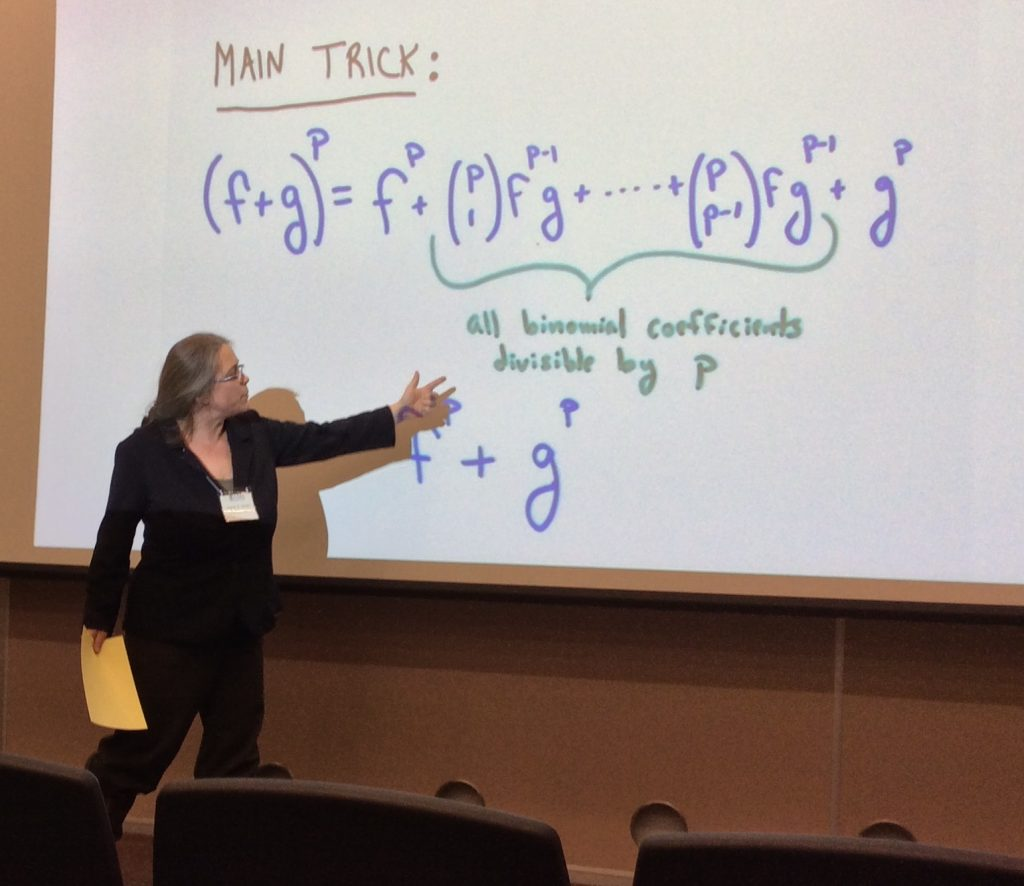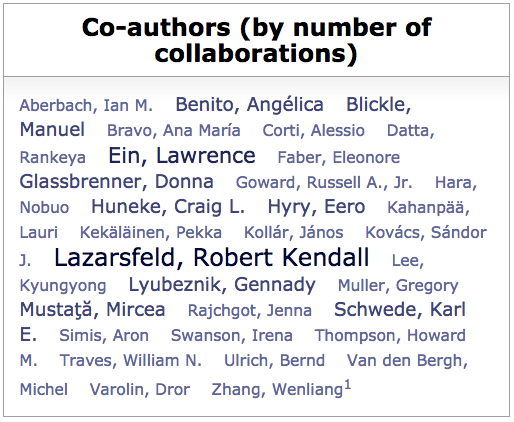# Karen SmithKaren Smith is a mathematician at the University of Michigan, which is where she also did her Ph.D.  Her thesis was on tight closure, an important topic in commutative algebra.  There is, of course,  a lot of overlap between commutative algebra and algebraic geometry, and Smith’s publications demonstrate this mix with about three quarters of them being in commutative algebra and a quarter in algebraic geometry.  Her publications are also a good demonstration of the collaborative nature of mathematics.

Smith seems to enjoy the communal aspects of mathematics.  She has organized conferences and two special years at MSRI.  She also has an excellent reputation as a teacher — to date, she has supervised 16 Ph.D. students —  and as a speaker.  This is a photograph of her as she gave a lively invited address at an AMS meeting in Albuquerque.  It is one of the few where she is not so animated as to be out of focus.   Smith is an active collaborator, having written papers and books with 32 different coauthors.   This is her coauthor cloud diagram on MathSciNet:Smith has written two books (both with coauthors) that are listed in MathSciNet:

MR2062787
Kollár, János; Smith, Karen E.; Corti, Alessio
Rational and nearly rational varieties.
Cambridge Studies in Advanced Mathematics, 92. Cambridge University Press, Cambridge, 2004. vi+235 pp. ISBN: 0-521-83207-1

MR1788561
Smith, Karen E.; Kahanpää, Lauri; Kekäläinen, Pekka; Traves, William
An invitation to algebraic geometry.
Universitext. Springer-Verlag, New York, 2000. xii+155 pp. ISBN: 0-387-98980-3

She has another:

Johdatusta algebralliseen geometriaan by Lauri Kahanpää, Karen E. Smith and Pekka Kekäläinen, which she describes as the Finnish version of An Invitation to Algebraic Geometry.

The second book is aptly titled, as the writing is engaging and, as our reviewer wrote, it “will recruit new enthusiasts” to the subject.

In an earlier blog post, I mentioned the cross-linking between MathSciNet and the MacTutor History of Mathematics Archive.  Smith is among the small group of living mathematicians to have a biography on the MacTutor site.  The Agnes Scott site of Biographies of Women Mathematicians also has a biography of Smith.

Let me close with a few reviews of Karen Smith’s work.

MR3352824
Benito, Angélica(1-MI); Muller, Greg(1-MI); Rajchgot, Jenna(1-MI); Smith, Karen E.(1-MI)
Singularities of locally acyclic cluster algebras.
Algebra Number Theory 9 (2015), no. 4, 913–936.
13F60 (13A35 14B05)

Cluster algebras were introduced by S. Fomin and A. Zelevinsky [J. Amer. Math. Soc. 15 (2002), no. 2, 497–529; MR1887642]. Locally acyclic cluster algebras, recently introduced in [G. Muller, Adv. Math. 233 (2013), 207–247; MR2995670], are a large class of cluster algebras which include many interesting examples from representation theory and Teichmüller theory.

In this paper, the authors focus on the properties of singularities of locally acyclic cluster algebras over an arbitrary field $k$. Let ${\mathcal A}$ be a locally acyclic cluster algebra. The main theorem is that ${\mathcal A}$ over an $F$-finite field $k$ of prime characteristic is strongly $F$-regular and ${\mathcal A}$ over a field $k$ of characteristic zero has $($at worst$)$ canonical singularities, where $F$ is the Frobenius endomorphism. In addition, they also show that the upper cluster algebra ${\mathcal U}$ determined by ${\mathcal A}$ is always Frobenius split.

On the other hand, when ${\mathcal A}$ is nonlocally acyclic, the authors prove the following result: If ${\mathcal A}$ is strongly $F$-regular then ${\mathcal U}$ is strongly $F$-regular. Moreover, they also provide examples which demonstrate that in this case the strong $F$-regularity of ${\mathcal U}$ is still possible, though not necessary.

Finally, the authors claim that the lower bound algebra is also Frobenius split and investigate the canonical modules of upper cluster algebras in the Appendix.

Reviewed by Yichao Yang

MR2068967
Ein, Lawrence(1-ILCC); Lazarsfeld, Robert(1-MI); Smith, Karen E.(1-MI); Varolin, Dror(1-IL)
Jumping coefficients of multiplier ideals.
Duke Math. J. 123 (2004), no. 3, 469–506.
14B05 (32S05)

The authors introduce new invariants, which are generalizations of the log canonical threshold in a natural way: Let $X$ be a smooth complex algebraic variety and let $x \in X$ be a fixed point. Given an effective divisor $A$ on $X$, for any rational number $c>0$, one can define the multiplier ideal ${\mathcal J}(X, c\cdot A) \subseteq{\mathcal O}_X$. Then there exists an increasing sequence$$0=\xi_0(A;x)<\xi_1(A;x)<\xi_2(A;x)< \cdots$$ of rational numbers $\xi_i=\xi_i(A;x)$ characterized by the following properties: ${\mathcal J}(X, c \cdot A)_x ={\mathcal J}(X, \xi_i \cdot A)_x$ for $c \in [\xi_i, \xi_{i+1})$, while $${\mathcal J}(X, \xi_{i+1} \cdot A)_x \neq{\mathcal J}(X,\xi_{i} \cdot A)_x$$ for every $i$. Here $\xi_1$ is the log canonical threshold of $A$ at $x$. The authors call these rational numbers $\xi_i(A;x)$ the jumping coefficients or jumping numbers of $A$ at $x$. The paper under review develops the theory of jumping coefficients and gives a number of applications.

In Section 1, several formal properties of jumping coefficients are established by taking advantage of geometric properties of multiplier ideals which the reader can find in R. K. Lazarsfeld’s book [Positivity in algebraic geometry. II, Springer, Berlin, 2004; MR2095472]. In Section 2, a result due to Kollár and others [B. Lichtin, Ark. Mat. 27 (1989), no. 2, 283–304; MR1022282; J. Kollár, in Algebraic geometry—Santa Cruz 1995, 221–287, Proc. Sympos. Pure Math., 62, Part 1, Amer. Math. Soc., Providence, RI, 1997; MR1492525; T. Yano, Publ. Res. Inst. Math. Sci. 14 (1978), no. 1, 111–202; MR0499664] concerning a relationship between the log canonical threshold and the Bernstein-Sato polynomial is generalized. Developing further the line of Kollár’s argument, the authors show that if $\xi$ is a jumping coefficient of $f \in \Bbb{C}[t_1, \dots, t_d]$ lying in the interval $(0,1]$, then $-\xi$ is a root of the Bernstein-Sato polynomial of $f$. In Section 3, the authors connect jumping coefficients to uniform Artin-Rees numbers, which were introduced by C. L. Huneke [Invent. Math. 107 (1992), no. 1, 203–223; MR1135470]. As a corollary, they prove that if $(f=0)$ has an isolated singularity at a point $x \in X$ but otherwise is smooth, then $\tau(f,x)+\dim X$ is a uniform Artin-Rees number for $f$, where $\tau(f,x)$ denotes the Tyurina number of $f$ at $x$. In the last section, the authors attach jumping coefficients to graded families ${\mathfrak a}_{\bullet}$ of ideals using the asymptotic multiplier ideals ${\mathcal J}(c \cdot{\mathfrak a}_{\bullet})$ and establish a few results on them. These invariants are not necessarily rational and they point out that the collection of jumping coefficient of ${\mathfrak a}_{\bullet}$ can contain cluster points, but it satisfies the DCC.

The jumping coefficients are closely related to several other invariants and shed a new light on singularity theory.

Reviewed by Shunsuke Takagi

MR1826369
Ein, Lawrence(1-ILCC); Lazarsfeld, Robert(1-MI); Smith, Karen E.(1-MI)
Uniform bounds and symbolic powers on smooth varieties.
Invent. Math. 144 (2001), no. 2, 241–252.
13A10 (13H05 14Q20)

The authors use the theory of multiplier ideals to prove several uniform bounds, the most remarkable of which are the uniform bounds for symbolic powers on smooth varieties. Namely, if $X$ is a non-singular quasi-projective variety defined over the complex numbers and $Z$ is a reduced subscheme of $X$, let ${\mathfrak q}$ be the ideal sheaf of $Z$. If all the irreducible components of $Z$ have codimension at most $e$ in $X$, then the authors prove that for all $n \ge 1$, ${\mathfrak q}^{(ne)}\subseteq{\mathfrak q}^n$. The key ingredients in this remarkable constructive result are the asymptotic multiplier ideals and the subadditivity property of multiplier ideals, the latter proved by J.-P. Demailly, L. M. H. Ein and R. K. Lazarsfeld [Michigan Math. J. 48 (2000), 137–156; MR1786484].

Inspired by the results of this paper, Hochster and Huneke extended this symbolic powers result to arbitrary Noetherian regular rings containing a field [M. Hochster and C. Huneke, “Comparison of symbolic and ordinary powers of ideals”, preprint, 2000; per bibl.]. Hochster and Huneke used the theory of tight closure, pointing to yet another connection between multiplier ideals and tight closure. (For other such connections [see, e.g., K. E. Smith, Comm. Algebra 28 (2000), no. 12, 5915–5929 MR1808611 ].)

The reviewer had proved that (under fewer assumptions) there exists an integer $l$ such that for all $n$, ${\mathfrak q}^{(nl)}\subseteq{\mathfrak q}^n$ [Math. Z. 234 (2000), no. 4, 755–775; MR1778408], but the construction there does not produce the integer $l$ even with the extra restrictions of either the paper under review or of the paper of Hochster and Huneke.

Ein, Lazarsfeld and Smith also prove the analogous uniform bounds when the family of symbolic powers of a radical ideal is generalized to any countable graded family of nonzero ideals $\{{\mathfrak a}_n\}$ satisfying ${\mathfrak a}_n\cdot{\mathfrak a}_m \subseteq{\mathfrak a}_{n+m}$ for all $n,m\ge1$. A special case is when ${\mathfrak a}_n$ is the contraction of the $n$th power of the maximal ideal of a Rees valuation (i.e., a prime divisor of the first kind), giving a constructive improvement of a restricted case of Izumi’s theorem [S. Izumi, Publ. Res. Inst. Math. Sci. 21 (1985), no. 4, 719–735; MR0817161], [D. Rees, in Commutative algebra (Berkeley, CA, 1987), 407–416, Springer, New York, 1989; MR1015531], or [R. Hübl and I. Swanson, J. Pure Appl. Algebra 161(2001), no. 1-2, 145–166 MR1834082 ].

Reviewed by Irena Swanson